# Betting Odds Converter – Easy Odds Conversion

Are you new to sports betting and feeling a bit confused by how to convert odds between all the different formats sportsbooks use? No worries at all! To figure out odds, there are many helpful odds converters like the one you’ll find in this article.

An odds converter shows you equivalent betting odds across multiple different formats for displaying odds. All equivalent odds pay equally in terms of your winnings. Our odds converter also shows the implied probability of an outcome according to the given odds.

Take a look at the table below for our betting odds converter. Read on and we’ll also explain how to convert odds using the table, as well as describe each type of betting odds. You’ll also learn a little about how to use implied probability to make informed wagers.

The content on this page is for informational purposes only. Vegas Insider makes no representation or warranty as to the accuracy of information given or the outcome of any game or event.

## ODDS CONVERSION TABLE

FractionDecimalAmericanImplied Probability
1/51.2-50083.3%
2/91.22-45081.8%
1/41.25-40080%
2/71.29-35077.8%
3/101.30-333.376.9%
1/31.33-30075%
4/111.36-27573.3%
4/91.44-22569.2%
5/42.25+12544.4%
11/82.38+137.542.1%
9/110.00+90010%
10/111.00+10009.1%
20/121.00+20004.8%
50/151.00+50002%
100/1101.00+100001%
1000/11001.00+1000000.1%

##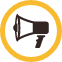WHY USE AN ODDS CONVERTER?

Just like you can probably balance your budget without a calculator, you could probably convert betting odds without an odds converter. But why wouldn’t you use a tool that can help you get the job done more efficiently and with more accuracy?

An odds converter gives you the simplest way for how to convert odds. Rather than working out the math yourself, the odds converter shows you how to read odds no matter how a sportsbook presents them.

Using an odds converter means you can always read odds in the format you’re most comfortable with. You can also convert to the odds format that you find easiest to use to calculate your winnings.

##BETTING ODDS CONVERTER - HOW TO USE

Looking at the table, you may not immediately see how to convert odds using an odds converter. But it’s relatively straightforward to read once you get used to it.

The columns of the betting odds converter show different formats for odds, as well as the implied probability. Odds that appear along the same row of the betting odds converter are mathematically equivalent, meaning that they will pay out the same.

Rows on the odds converter also tell you what the implied probability of each set of odds is. Seeing the implied probability helps you bet wisely. You’ll know how likely a sportsbook feels an event is. There’s more information on the implied probability to come in the following section.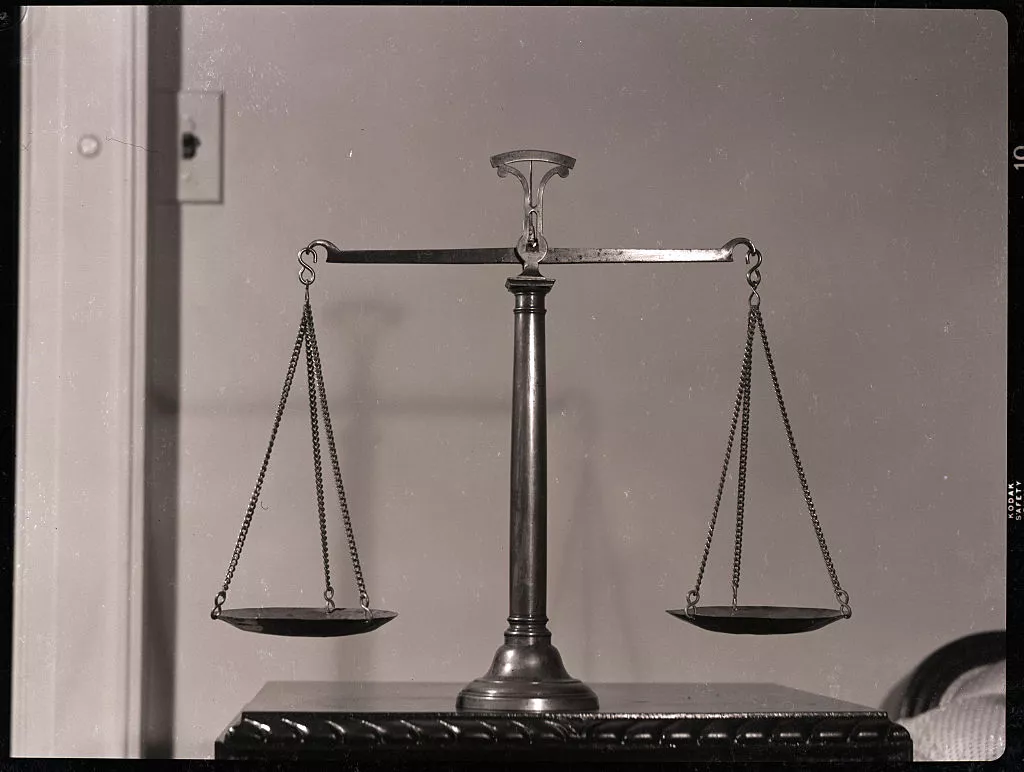They're all telling you the same information, but you may need to convert the odds on your preferred website. Use this odds converter! Getty

## UNDERSTANDING THE DIFFERENT ODDS FORMATS

You’ll come across a number of different formats when browsing offers from sportsbooks. You can use the betting odds converter to figure out what these odds mean no matter how they’re formatted.

### AMERICAN

You may also see these odds called moneyline odds, Vegas odds, or US odds. American odds appear as either a positive or negative three-digit number. The favorite is listed with a negative number, while the underdog has a positive number for betting odds. The odds converter shows both types, starting from negative and going up to positive.

Positive odds that you see on the betting odds converter tell you what profit you would earn for betting \$100. For example, if the Dolphins are +150 to win a game, you would receive \$75 in profit for a correct \$50 wager. This would pay out a total of \$125.

Negative odds show what a bettor needs to wager to earn \$100 in profit. So if the Rams have -200 odds to win a game, then a bettor would need to wager \$200 to make \$100 in profit. This would give them a total of \$300.

### DECIMAL

Decimal odds are some of the most straightforward to read of all the other formats shown on the odds converter. They display as a number followed by a decimal point. This number indicates the potential return for every \$1 wagered.

For example, if Lebron James had .60 odds to win the MVP award for the season, you would receive \$0.60 for every dollar you bet if you predicted correctly. A \$100 bet in this example would payout a total of \$160.

### FRACTIONAL

Fractional odds are written to show how many times a sportsbook predicts an outcome will theoretically succeed or fail. The first or top number shows how many times the outcome is predicted to succeed, while the second or bottom number shows how many times it will fail.

For example, if the Yankees are given 1/2 odds to win a game, the sportsbook is predicting that they will bring home the win two times out of three. They are favorites in this example scenario, so they won’t pay as well for a successful wager as their opponents.

You can also use the odds to calculate your winnings. For our example, a correct wager on 1/2 odds pays \$1 for every \$2 bet. So if you bet \$100, you could expect a profit of \$50 on a correct wager, for a total payout of \$150.

## WHAT IS IMPLIED PROBABILITY?

Reading between the lines on odds can tell you how likely a sportsbook calculates an event to be. This is called the implied probability of an outcome. Some sportsbooks have access to expert analysis and data to determine this implied probability, while others simply follow the market to determine their odds.

You can make informed bets if you figure out the probability. If you feel an outcome has a better chance of occurring than what the sportsbook has calculated, you can maximize your chance of placing a high-value wager.

Each type of odds explained above has a different formula for determining implied probability. Lucky for you, our betting odds converter shows you how to convert odds into an implied probability for any odds you might come across.

### HOW DO I CALCULATE IMPLIED PROBABILITY, AND WHY DOES IT MATTER?

You can use the above converter to calculate implied probability. The math behind this is pretty simple. It will differ, however, whether you're trying to convert decimal odds, fractional odds, or American odds. You ignore the positive and negative sign when calculating these odds. For the sake of explanation, let's look at converting negative American odds:

odds / (odds + 100) * 100 = implied probability

Therefore, if you're looking at a bet set at -500 odds, that means that outcome has about an 83% chance of happening. 500/(600) = 5/6 * 100 = 83.3%. Hence, the more likely outcome indicates the favorite to win a certain bet. The opposite math is true for positive odds:

100 / (odds + 100) * 100 = implied probability

If, for example, you're looking at a +450 bet, that means the outcome is calculated to have about an 18% chance of winning. Sometimes, looking at the actual percentages by calculating implied probability helps you make a smarter bet, so it's a good idea to know how to calculate it.

##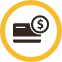WHERE TO PLACE A BET AFTER CONVERTING YOUR ODDS?

Here is our expert selection of the bookmaker where you can place a great sports Bet right away :

Book
Offer
Legal States

*In-person registration and betting only in MS.
**The BetMGM app is available for mobile wagers in Washington DC, but only at Nationals Park.

Updated on : 11/30/2023

##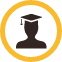FAQ

Here’s a quick reference for any questions you may have about the different odds formats or how to convert odds.

### WHAT IS THE MOST COMMON FORMAT FOR BETTING ODDS?

The most common betting odds format you’ll see depends on the type of sport. Football favors American odds, while international events like tennis tournaments or soccer matches are more likely to use decimal odds. UK sportsbooks prefer fractional odds, especially for horse races.

Different sportsbooks will also have different standards for what odds format they show for particular sports. No matter what type of format you see, using an odds converter can help you make sense of the odds that are offered.

### HOW DO YOU CONVERT ODDS TO PROBABILITY PERCENTAGES?

Experienced sports bettors can figure out what probability a sportsbook has calculated for a particular outcome. This is done by converting odds into percentages. Another name for this percentage is the implied probability.

It’s easiest to see this conversion with fractional odds. An outcome with 1/5 odds is expected to occur 5 out of every 6 times, or 83.3% of the time. Meanwhile, an outcome with 20/1 odds is only expected to occur once every 21 theoretical outcomes. This converts to 4.8%.

These calculations may seem complicated, but using an odds converter makes it easy. You can see the probability percentage for any odds format at just a glance. That helps you make informed bets based on what outcome the sportsbook’s data is predicting.

### HOW DO I FIGURE OUT MY POTENTIAL PAYOUT?

All of this information about odds converters, probability percentages, and different formats of odds is helpful, but you’re probably wondering how much you get paid. Each different type of betting odds have a different formula for calculating your winnings.

Perhaps the most straightforward equation for calculating your winnings uses the decimal format. Here you would simply multiply your stake by the odds given, then subtract your stake from the result.

There are other formulas for calculating your winnings from the other odds formats. Or you can use an odds converter to convert to decimal odds and follow the steps above. Using an odds converter lets you handle the calculations in the way that is most comfortable for you.

##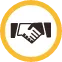FINAL THOUGHTS

Sports betting odds can be displayed in a variety of formats. Using an odds converter allows you to convert odds to the format you find easiest to understand. That has the added benefit of making it easy to calculate your winnings no matter how a sportsbook is displaying their odds.

Some odds converters, like the table in this article, also give you the implied probability percentage for any given odds. This is important because it helps you understand how likely a bookie predicts an outcome to be. Understanding how the bookies are thinking is the first step to becoming a successful sports bettor.

All that time you save on converting odds is more time you can spend watching the game and playing with your favorite sportsbooks. Refer to our helpful guide anytime you need pointers on how to read the offers from a sportsbook and you’ll be able to find the most competitive offers.

Other great calculators you can use to choose your best possible returns:

Arbitrage Calculator | Betting Odds Calculator | Hedge Calculator | Moneyline Calculator | Teaser Calculator